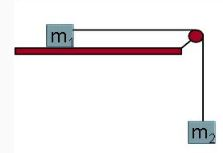# A mass m1 = 5.4 kg rests on a frictionless table and connected by a massless string over a...

## Question:A mass m1 = 5.4 kg rests on a frictionless table and connected by a massless string over a massless pulley to another mass m2 = 5.7 kg which hangs freely from the string. When released, the hanging mass falls a distance d = 0.7 m.

1) How much work is done by gravity on the two block system? J

2) How much work is done by the normal force on m1? J Your submissions: 0 Computed value: 0 Submitted: Sunday, October 22 at 5:30 PM Feedback: Correct!

3) What is the final speed of the two blocks?

4) How much work is done by tension on m1?

5) What is the tension in the string as the block falls?

6) The work done by tension on only m2 is:

7) What is the NET work done on m2?

## Newton's Second Law

According to Newton's second law, the net force on an object is given by the product of mass and acceleration of the object. If the object is at rest then force on it would be zero.

## Answer and Explanation:

(1)

Work done by gravity is equal to the change in potential energy. Therefore

{eq}\displaystyle W = m_{2}gd \\ W = 5.7*9.81*0.7 \\ W = 39.14 \ J {/eq}

(2)

Since there is no motion in the vertical direction, therefore the work done will be ZERO.

(3)

Now, let us consider that the blocks move with an acceleration of "a"

Therefore applying Newton's Second law on mass 1

{eq}\displaystyle T = m_{1}a {/eq}

where

• T is the tension in the string

Now for the mass 2

{eq}\displaystyle m_{2}g - T = m_{2}a \\ m_{1}a + m_{2}a = 5.7*9.81 \\ ( m_{1}+m_{2} ) a = 55.92 \\ a (5.4+5.7) = 55.92 \\ a = 5.04 \ m/s^{2} {/eq}

Now Using the kinematic equation

{eq}\displaystyle v^{2} = u^{2} + 2ad \\ v^{2} = 0 + (2*5.04*0.7) \\ v = 2.66 \ m/s^{2} {/eq}

(4)

Now the tension in the string would be

{eq}\displaystyle T = m_{1}a \\ T = 5.4*5.04 \\ T = 27.22 \ N {/eq}

Now the work done will be

{eq}\displaystyle W_{1 } =T*d \\ W_{1} = 27.22*0.7 \\ W_{1} = 19.05 \ J {/eq}

(5)

Tension in the string is 27.22 N

(6)

Work done on mass 2 will be

{eq}\displaystyle W_{2 } =T*d \\ W_{2} = 27.22*0.7 \\ W_{2} = 19.05 \ J {/eq}

(7)

Now the net work done on mass 2

{eq}\displaystyle W_{net} = (mg-T)*d \\ W_{net} = [ (5.7*9.81) - 27.22 ]* 0.7 \\ W_{net} = 20.09 \ J {/eq}

#### Learn more about this topic:Newton's Laws of Force & Motion

from GED Science: Life, Physical and Chemical

Chapter 7 / Lesson 6
9.6K